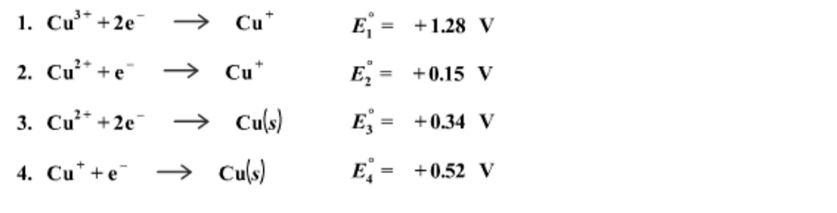Problem: Given the following half-reactions and their respective standard reduction potentials calculate the standard reduction potential for the reduction half-reaction of Cu(lll) to Cu(ll).Cu3+ + e- → Cu2+

FREE Expert Solution
96% (127 ratings)
Problem Details

Given the following half-reactions and their respective standard reduction potentials calculate the standard reduction potential for the reduction half-reaction of Cu(lll) to Cu(ll).

Cu3+ + e- → Cu2+What scientific concept do you need to know in order to solve this problem?

Our tutors have indicated that to solve this problem you will need to apply the Cell Potential concept. If you need more Cell Potential practice, you can also practice Cell Potential practice problems .

What is the difficulty of this problem?

Our tutors rated the difficulty of Given the following half-reactions and their respective stan... as high difficulty.

How long does this problem take to solve?

Our expert Chemistry tutor, Dasha took 10 minutes to solve this problem. You can follow their steps in the video explanation above.

What professor is this problem relevant for?

Based on our data, we think this problem is relevant for Professor Geissler's class at CAL.